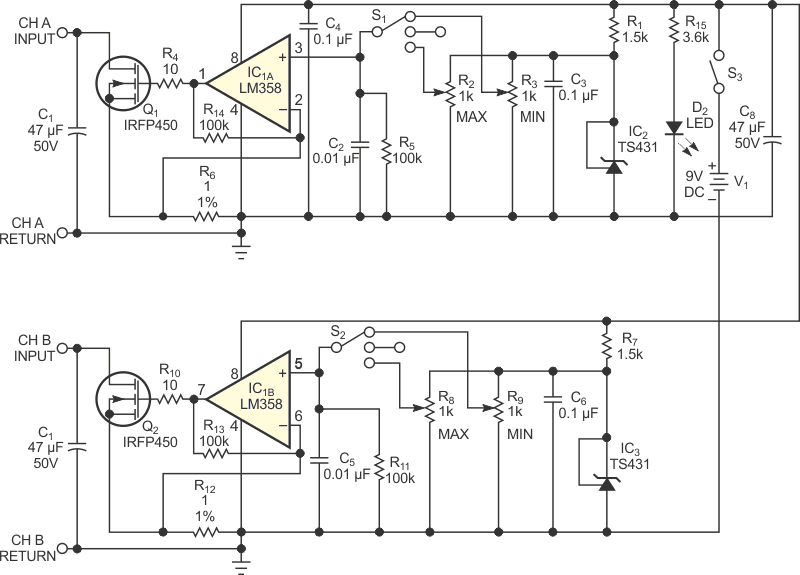# Simple dual constant-current load tests low-current power supplies

## Texas Instruments LM358

Today’s small electronic appliances, such as washers, dryers, and stoves, use switched-mode power supplies to replace bulky, heavy, linear-power supplies. The engineer testing these power supplies, which range in current from 50 mA to 1 A, typically uses resistors or standard off-the-shelf electronic loads. An engineer would employ a variety of high-wattage resistors to verify multiple loading conditions to satisfy a proper design. Most off-the-shelf electronic loads target an average of 300 W. When measuring 50 to 300 mA, a display is inaccurate; most of them display 0.1 A, but accuracy is questionable at that low range. You can alternatively use the simple dual constant-current-load design in Figure 1, which you can build with inexpensive, common parts.Figure 1. This dual constant-current load can measure the performance of dual power supplies supplying 0 to 1.25 A per channel.

The load current passes through a MOSFET and a 1%, 1 Ω sense resistor, R6. Pin 2 of IC1A compares the voltage drop in the resistor to a reference voltage. IC1, an LM358 op amp, compares the two inputs and adjusts its output accordingly. The reference voltage at Pin 3 of IC1A comes from a voltage-divider potentiometer, R2 or R3, which derives from a TS431 1.25 V 1% reference. Because the maximum voltage can be 1.25 V and the sense resistor’s value is 1 Ω, the maximum current per channel can reach 1.25 A.

R2 and R3 are 15-turn, 1-kΩ potentiometers, which you can finely adjust to the desired load. One can set a minimum current, and the other can set a maximum current. Switch S1 can then switch between minimum load, no load in the middle position, and maximum load. Furthermore, by attaching a standard DMM (digital multimeter) across R6, you can directly read the current and adjust it to the proper level.

Input-voltage change does not affect the DMM’s reading because it monitors the constant current through sense resistor R6. The second channel is a duplicate of the first. Each channel can control 0 to 1.25 A and can handle a voltage of 3 to 50 V. The capacitor input and the MOSFET set the upper limit. The two inputs can be in parallel to a load of 2.5 A. For a two-output power supply, you can set the minimum and maximum current by precisely reading the level on a multimeter and then quickly testing a matrix of no load, minimum load, and maximum load. A 9 V battery powers the unit.

EDN